Next: Experimental Results Up: Analysis of the Particle Previous: Conductivity Estimation

## Ionization Rate Estimation

A method primarily used to determine emission features of the auroral spectrum can be used to test the effects of electron precipitation on the ionosphere at F-layer heights, namely ionization rate estimation. Using a model of the angular distribution for the primary electron stream, one can compute ionization rate height profiles from a given energy spectrum. This ionization rate can serve as an indicator for modification of the ionospheric conditions by the electron precipitation for heights from 100 to 500 km, i.e. most importantly at F-layer heights above 180 km.

The actual formula used for this thesis is based on original work by Rees [Ree63]. An empirical function for the energy absorption of an electron beam in matter was derived by Lazarev [Laz65] and related to the energy distribution of an electron beam as it travels through air layers of different mass. A formula was obtained for computing the ionization rate at various levels in the upper atmosphere during injection of electron beams of various energies. The Lazarev model has advantages over that of Rees, since it requires knowledge only of density, depth, energy and flux and is simpler to calculate. The ionization rate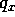at some depth x (corresponding to the penetrated mass) is given by the following expressions: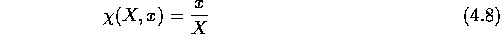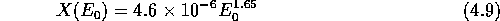where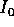is the intensity of the electron beam,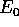is the energy of the electrons in the beam,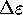is the average energy necessary to achieve a single ionization (34 eV for air),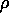is the density of the medium. Since x andare height dependent, an atmospheric model can be used to give estimates of their values over a broad range of heights. Thus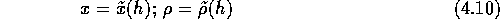In the actual calculations, density and depth profiles derived from the MSIS86 model were used.To estimate the total ionization rate due to a particular energy spectrum at a given height h, one has to simply integrate over all energy channels available: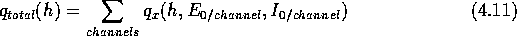More recently, the occurrence of F-layer patches due to soft-electron precipitation has been confirmed by Watermann et al. [WdN92, WLd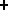94]. These comparisons of incoherent scatter radar and DMSP satellite measurements show patches produced by the direct entry of magnetosheath electrons into the magnetosphere followed by precipitation into the upper F-layer. Nakajima et al. [NFRO93] observed suprathermal electron bursts at low energies that are very localized (approximately 20-30 km along the satellite path at satellite altitudes of) .Next: Experimental Results Up: Analysis of the Particle Previous: Conductivity Estimation

Andreas Schiffler
Wed Oct 9 10:05:17 CST 1996